# SAT Math Multiple Choice Question 810: Answer and Explanation

### Test Information

Question: 810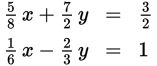15. If the ordered pair (x, y) satisfies the system of equations above, what is the sum of the values of x and y?

• A.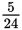• B.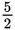• C.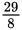• D.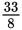Explanation:

D

Difficulty: Hard

Category: Heart of Algebra / Systems of Linear Equations

Strategic Advice: When a system of equations involves lots of fractions, multiply each equation by its least common denominator to clear the fractions.

Getting to the Answer: Multiply the first equation by 8 and the second equation by 6 to clear the fractions: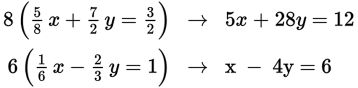Now, examine the new coefficients. Notice that multiplying the second equation by 7 (which yields 7x - 28y = 42) and adding the two equations will eliminate the y terms, leaving a single equation with one variable: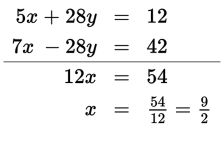Substitute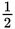for x into the easier of the modified equations (the equations without the fractions), and solve for y: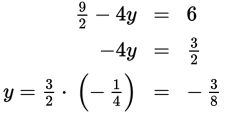The question asks for the sum of x and y, so add to get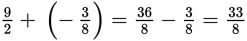, which is (D).